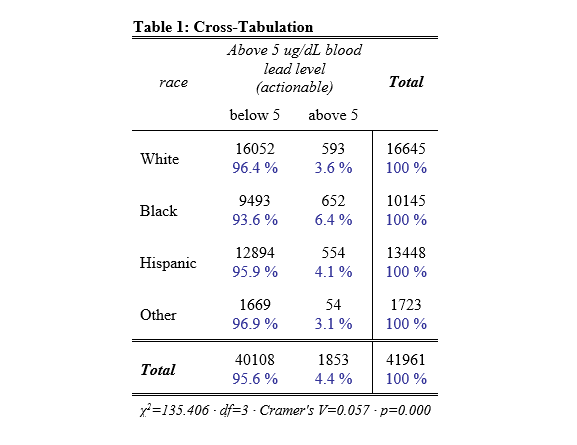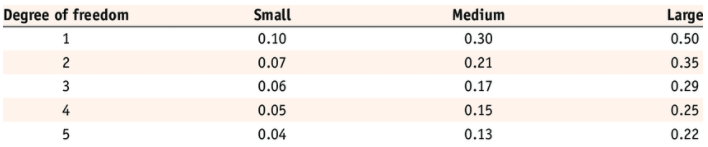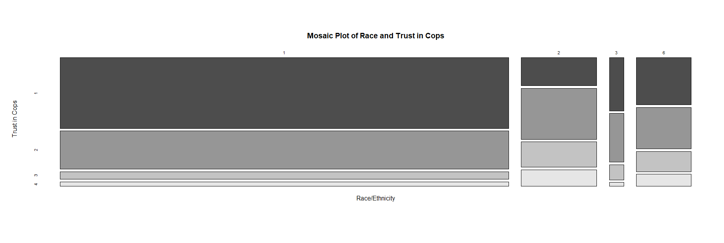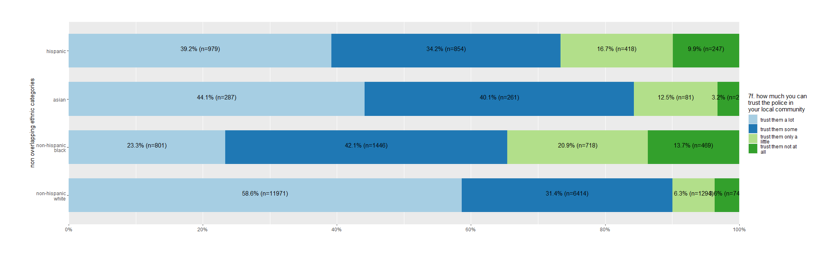# Chapter 8 Cross-Tabulation

This chapter provides generic code for generating a contingency table and carrying out a chi-square test of independence. It is recommended that you proceed through the sections in the order they appear.

Placeholders that need replacing:

• mydata – name of your dataset
• rowvar – name of your row variable (usually your causal factor)
• object – whatever you want to call your object(s))
• label – any titles, axis labels, category labels

## 8.1 Packages Needed for Cross-Tab

This code will check that required packages for this chapter are installed, install them if needed, and load them into your session.

``````req <- substitute(require(x, character.only = TRUE))
libs<-c("sjPlot")
sapply(libs, function(x) eval(req) || {install.packages(x); eval(req)})``````

## 8.2 Data Prep for Cross-Tab

I recommend consulting the resources on knowing your data and data prep. You may want to add category labels and potentially collapse categories.

## 8.3 Cross-Tabulation, Chi-Square Test of Independence, and Effect Size

The sjPlot package allows us to conduct the cross-tabulation and chi-square test of independence at the same time using its tab_xtab() function. The command produces a html contingency table, chi-square test results, and Cramer’s V statistic.

``sjPlot::tab_xtab(var.row = mydata\$rowvar, var.col = mydata\$colvar, title = "Table Title", show.row.prc = TRUE)``Interpretation of Cramer’s V is contingent on the degree of freedom. The following table offers guidance on the appropriate characterization of V.## 8.4 Graphing Options for Cross-Tab

A table is often your best bet for representing a contingency table. Beyond the table, you might consider your graphing options, which include a mosaicplot or sjPlot’s bar graph plot.

I also encourage you to check out the R Graph Gallery, a website that showcases different graphs and provides their associated code.

In this series of commands, the first command generates an object containing the cross-tab results. The mosaicplot command then translates that object into a mosaic plot.

``````object <- table(mydata\$rowvar, mydata\$colvar)
mosaicplot(object, main = "Title of Graph", xlab = "X-axis label", ylab = "Y-axis label", color = TRUE)``````Alternatively, the sjPlot package allows us to generate a stacked bar chart.

``sjPlot::plot_xtab(mydata\$rowvar, mydata\$colvar, margin = "row", bar.pos = "stack", coord.flip = TRUE)``## 8.5 Consolidated Code for Cross-Tab

Below is the consolidated code from this chapter. One could transfer this code into an empty RScript, which also offers the option of find/replace terms. You can also download the generic cross-tab RScript file here.

Placeholders that need replacing:

• mydata – name of your dataset
• rowvar – name of your row variable (usually your causal factor)
• object – whatever you want to call your object(s))
• label – any titles, axis labels, category labels
``````# 7.1   Packages Needed

req <- substitute(require(x, character.only = TRUE))
libs<-c("sjPlot")
sapply(libs, function(x) eval(req) || {install.packages(x); eval(req)})

# 7.2 Know your data and prep as needed

# 7.3 Conduct the cross-tabulation and chi-square test at the same time using sjPlot’s tab_xtab() function,
#       which (produces html contingency table, chi-square test results, and Cramer’s V)

sjPlot::tab_xtab(var.row = mydata\$rowvar, var.col = mydata\$colvar, title = "Table Title", show.row.prc = TRUE)

# 7.4 Graphing Options

## A table is often your best bet for representing a contingency table. Beyond the table,
## you might consider your graphing options, which include a mosaicplot or sjPlot’s bar graph plot.

## mosaicplot

object <- table(mydata\$rowvar, mydata\$colvar)
mosaicplot(object, main = "Title of Graph", xlab = "X-axis label", ylab = "Y-axis label", color = TRUE)

## sjPlot’s plot_xtab() function
sjPlot::plot_xtab(mydata\$rowvar, mydata\$colvar, margin = "row", bar.pos = "stack", coord.flip = TRUE)
``````